## Monday, November 24, 2008

### Secant and TangentThe names of the trigonometric ratios tangent and secant are derived from the Latin “to touch” and “to cut” – the tangent to a figure is a line that touches it in one place, where a secant cuts through it in two or more. But how are these geometric terms related to the ratios that bear their names? The answer can be shown using the diagram at the top of the post - a diagram that used to be a standard one in high school trig text books.

Consider the acute angle BAC. Allow |AC| = 1, and construct a unit circle about A that goes through C. Construct a tangent to this circle at C, and extend the segment AB so that it meets this tangent at E. So, the segment CE lies on the tangent while the segment AE lies on the secant of the unit circle formed around BAC. ACE is a new right triangle that contains the original BAC.

The tangent of BAC is BC/AB (opposite/adjacent), but if we now look at the second triangle ACE, we see tht it is also given by (CE/AC)=(CE/1)=CE - the tangent is measured by the segment of the tangent, CE. Similarly, the secant of BAC is given by AC/AB (hypoteneuse/adjacent), but again turning to the second triangle ACE, we see that this is (AC/AB)=(AE/AC)=(AE/1)=AE - and the secant is provided by the length of the secant, AE.

This treatment was taken from the book "Plane Trigonometry and Tables" by G. Wentworth, published in 1903. In some of the texts of this era, the "primary" trigonometric ratios were sin, sec, and tan (rather than sin, cos, and tan), perhaps owing their primacy to constructions like the one described above.

The cosine was considered a secondary trigonometric ratio - its name coming from the phrase "complement's sine." Along with the usual ratios, texts often presented several convienience ratios that are now antiquated, such as the versedsine vrsin(x) = 1-cos(x) and the half-versed sine or haversine hvrsn(x)= (1/2)vrsin(x).

The most fundamental trigonometric ratio has the most obscure name. It is generally claimed that the word “sine” comes from Latin word for “bend,” but some have suggested that the word is ultimately derived from the name of the curve formed by the gathering of a toga, or from the Latin word for “bowstring.” In Arithmetic, Algebra, Analysis, Felix Klein states that “sine” represents a Latin mis-translation of an Arabic word, but does not go on to explain its origins any further.

My edition of Wentworth is from 1903 - a PDF of the 1887 edition can be downloaded here.

## Monday, November 17, 2008

### Metaphors and Mathematics 3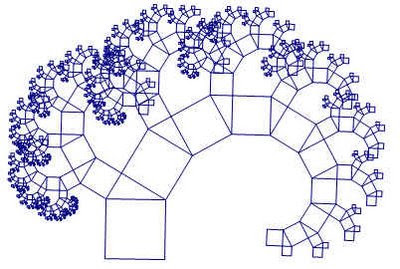In many traditions, Biedermann's Dictionary of Symbols tells us, "the tree was widely seen as the axis mundi around which the cosmos is organized" and, as mentioned in a previous post, has been widely used to describe the relationship between mathematics and other sciences. Mathematics itself, like many subjects, is often portrayed as a tree whose sub-topics make up branches that continue to grow and bifurcate.

Some recent articles have take a more postmodern perspective on using the tree metaphor to describe mathematics.

Dan Kennedy 's "Climbing around on the Tree of Mathematics," (full text here) and Greg McColm's "A Metaphor for Mathematics Education" are two recent articles that make arguments by analogy about what mathematics is and how it should be taught. In Kennedy's argument, mathematics is a tree, while in McColm's it is a vine - both are organic, growing, and branching. What distinguishes these two uses of metaphor from traditional tree analogies is that both authors are not at all suggesting that we can stand back and survey the structure as a whole and understand how all its parts are related. The ability to provide a comprehensive view of the subject, to make it surveyable, was the raison-d'être of metaphors like the "Tree of Science." Instead of using the metaphor this way, both authors suggest that we think of ourselves as part of the growing structure - as climbers and gardeners who cannot see the complex organic whole, but who can explore and tend to our small part of it. In these descriptions, natural forms like trees and plants, once metaphors for simplicity and comprehensibility, now provide metaphors for complexity.

Up in the Tree of Mathematics, Kennedy suggests that working mathematicians are labouring at extending its outer branches. This is where the view is best, where the fruit is found, and where the beauty of mathematics can be seen most clearly. School Mathematics is part of the trunk, the solid, oldest, stable part of the tree, and math teachers spend their time helping students climb the trunk, hoping that some may one day reach its outer branches. Unfortunately, the difficulty of the trunk prevents most people from ever climbing beyond it. Kennedy suggests that we should be less concerned with the trunk than with the branches, and that technology can provide a ladder to assist the climb.

McColm's Mathematical Vine is not mathematics itself, but a structure that clings to the underlying reality of mathematical truth. Mathematics, in this analogy, is like a hidden tower, whose shape can only be seen by looking at the vine that has taken shape around it. Like in Kennedy's analogy, working mathematicians are the caretakers who help the structure grow. For McColm, this analogy emphasizes the importance of mathematics education - a process of strengthening the vine so that it may continue to grow. Perhaps because his audience is primarily post-secondary researchers, he does not advocate finding shortcuts to "higher" views, but rather suggests that education be promoted through "tending to the vine" - clarifying mathematics and strengthening connections between different branches.

Although they suggest more of a structure at play, rather that a stable unified whole, organic metaphors like those used by McColm and Kennedy continue to suggest a natural unity among the various parts of mathematics. In that sense they are still rooted (or centered), and, although they have somewhat destabilized the tree analogy, they haven't quite deconstructed it. They have not, for example, gone quite as far as Wittgenstein, who seemed to suggest that metaphors that attempt to link the subjects of mathematics in a defining way like this are misguided. In his view, as described by Ackerman (1988, p. 115):
mathematics is an assemblage of language games, having no sharp and uniform external boundary, with potentially confusing and criss-crossing subdisciplines held together by an internal network of analogous proof techniques.
It is easy to appreciate how some climbers in Kennedy's trees and McColm's vines end up like the protagonist in Roz Chast's cartoon "Falling off the Math Cliff", where step 1 is "A boy begins his wondrous journey," and step 8 is "The plummet."

## Monday, November 10, 2008

### A Bit More on Factors and Extended Multiplication Tables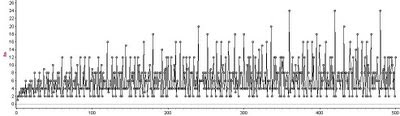As described in earlier posts, factor lattices and the extended multiplication table have a common link in an arithmetic function that we'll just call f.In the formula above, the p_i values are distinct primes. The function f tells us the number of times n appears in the extended multiplication table, and tells us the number of nodes in n's factor lattice. Note that f(1) = 1, and f(p) = 2 for every prime number p.

We can see from its formula that f(n) is multiplicative - if n and m are relatively prime, then f(nm) = f(n)f(m). This fact makes it easier to calculate f(n) values.

Other multiplicative arithmetic functions include the Euler totient function, theta(n), and the function that sums the divisors of n, sigma(n).

The graph at the top of this post shows the first 500 values of f(n).

## Thursday, October 23, 2008

### Metaphors and Mathematics 2Robert Recorde, author the first English textbook on algebra (published in 1557), chose to give his book the metaphorical title The Whetstone of Witte to encourage people to take up the new and difficult practice of algebra. The metaphor of a whetstone, or blade-sharpener, suggests that algebra is not only useful, but also good mental exercise. In the verse that he included on its title page, he writes,

Its use is great, and more than one. Here if you lift your wits to wet, Much sharpness thereby shall you get. Dull wits hereby do greatly mend, Sharp wits are fined to their full end.

Mathematics, and algebra in particular, according to The Whetstone of Witte is like a knife-sharpener for the brain. Four hundred years later, in his book Mathematician's Delight (1961), W.W. Sawyer takes up a similar metaphor, suggesting that "Mathematics is like a chest of tools: before studying the tools in detail, a good workman should know the object of each, when it is used, how it is used." Whether they describe mathematics as a sharpener or other tool, these mechanical metaphors are commonly used to emphasize the practicality and versatility of mathematics, particularly when employed in engineering or science, and suggest that it should be used thoughtfully, and with precision.

An often quoted mechanical metaphor that suggests a more frantic and less precise process of mathematical creation is often attributed to Paul Erdos: "a mathematician is a machine for turning coffee into theorems."

The "series" of posts on this is:
Metaphors and Mathematics 1
Metaphors and Mathematics 2
Metaphors and Mathematics 3

## Tuesday, October 21, 2008

### Metaphors and Mathematics 1When asked to describe mathematics we often resort to metaphor rather than attempt to provide strict definitions. These pictures from high school math textbooks from the 1930s are an example of this tendancy.

The simple hierarchies of these images resolve the complicated relationship between mathematics and science by appealing to our desire for an organic unity among disciplines, giving mathematics a foundational role within the general concept of science. These images are appealing, but do not stand up to scrutiny.

The simple relationship between mathematics and science becomes complicated when mathematics is described, as it sometimes is, as a science itself. It's definition as "the science of space and quantity" is further complicated by the caveat that it is an exact deductive science, unlike the usual inductive kind. Following this line of thinking further, mathematics is then described as a kind of meta-science, or a limit point to which science might aspire - science emptied of all of its empirical content, a science of pure thought. While some view mathematics as a foundation for science, others as a supra-science, the emerging field of experimental mathematics brings mathematics back into the empirical fold, reducing it (or elevating it) to a science like any other. So, mathematics can be seen as root, branch, or even the form of the tree itself.

Thinking about these things for even a short while evokes some sympathy with Bertrand Russell's remark that "mathematics may be defined as the subject in which we never know what we are talking about, nor whether what we are saying is true."The "series" of posts on this is:
Metaphors and Mathematics 1
Metaphors and Mathematics 2
Metaphors and Mathematics 3

## Wednesday, September 24, 2008

### Window Patterns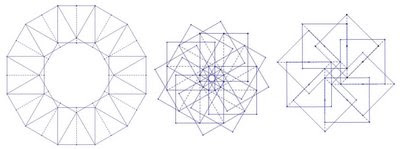Start with a square piece of paper (like a Post it note), fold and unfold it in half along a mid-line or along a diagonal. Take another identical square, and fold and unfold it the same way. Decide on some way to place the second square on the first, so that the second is somewhat rotated. Use only the edges of the square and the creases that you made to determine the placement. Make your placement precise so that your "rule" can be described exactly in terms of the edges and creases. Repeat this process, placing a third square on top of your second square using exactly the same rule. Repeat until your placing of papers leads you back to the first piece.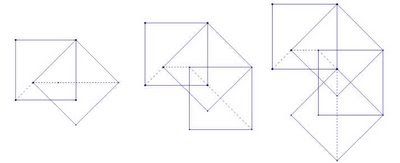The resulting construction might look something like the one shown on the left below. If you take your papers, set them in place with some careful light gluing, and place them on a window, the sunlight passing through the overlapping papers creates a stained-glass effect that shows a variety of shapes.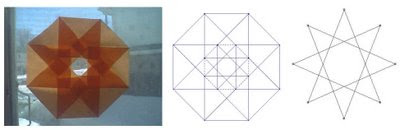This sort of construction is a simplified version of what William Gibbs describes in his book "Window Patterns." In Gibbs' treatment, the pattern is partially planned in advance, and then the dimensions of the rectangular pieces of paper that make up the pattern are determined using a little trigonometry. This process can be simplified by starting with a more limited range of options for paper dimension and placement. It turns out that a surprising number of window patterns can be created by only using squares, their mid-lines, and their diagonals, and that these patterns invariably have "special triangles" and related regular polygons and star-polygons embedded within them.

Here are a two more "placement rules" and the patterns that they give rise to.The diagrams were created using Geometer's Sketchpad - if you construct the rule using translations applied to a constructed square, you can use the iteration feature to create the final pattern. GSP provides a good environment for planning out the patterns prior to constructing them with paper, and building the plans in GSP is enjoyable and instructive as well.

## Friday, September 19, 2008

### Star PolygonsStarting with p (p a positive integer) equally distributed dots (vertices) around a circle. Connecting each dot to the next as you move around the circle will give you a regular p-gon - a p-sided polygon with p vertices. If, however, instead of connecting each dot to the one next to it you skip over a fixed number of dots, then you might end up with a star-like pattern, like the ones shown above. In this process, imagine that you start with a particular vertex and move in a counter-clockwise direction. If there are any dots left-over when you get back to the dot you started from, just throw away the unconnected dots.

The Schläfli notation for polygons is very useful for describing regular connected star polygons, and provides an example of how sometimes calculations with notation match exactly with calculations done with diagrams. In this notation, regular polygons like triangle, square, pentagon, etc. are written as {3}, {4}, and {5} respectively. A regular p-gon is written as {p}. If when drawing your p-gon you connect to the second next vertex instead of the first, then you would write this as {p/2}. If you connect to the q'th next vertex, then you would write this polygon as {p/q}. Note that if you are just connecting to the next vertex to make a regular p-gon, this notation gives you {p/1} = {p}, as you would expect.

If you start playing with this process you will notice that {p/q} gives you the same polygon as {p/(p-q)} (as long as you ignore the orientation of the polygon). You may also notice that if q is larger than p, you end up repeating the same patterns, in particular {p/q} = {p/(q mod p)}. Also you will notice that if p and q have common factors, you end up having skipped vertices. In our process we are throwing these away to ensure that our polygons are connected, but you can extend the process and keep them (see note below).

The process described is straightforward to implement in a program. The images shown here were generated in Tinkerplots. To implement it in Tinkerplots, you need two sliders - p and q, and the following attributes:

n = caseIndex()
theta = 2*n*pi(1-q/p)
x = cos(theta)
y = sin(theta)

If you create a plot with y vertical and x horizontal, choose "show connecting lines" and add a filter n<=p+1, you can add a large number of cases to the collection (~200, say) and be able to slide p and q to create a wide variety of connected star polygons. The only restriction is that p must be less than the number of cases you have created. There is nothing special about using Tinkerplots here - any programming environment with reasonable graphics should do a reasonable job (Logo would be fine. :)).

The polygons below are the regular connected polygons based on 12 vertices. Because 12 is divisible by 2, 3, 4, and 6 we end up with regular polygons triangle {3}, square {4}, hexagon {6} and only one star polygon {12/5}. The "degenerate" polygon {2} is known as a "digon." Here, drawing the diagram first and then seeing what polygon comes out will give you the same result as dividing p/q first and then drawing the corresponding polygon. In this sense, the notation and diagrams nicely reflect each other.Contrast this with the family of star polygons that are generated when a prime number of vertices are used. The images below are the family of regular connected polygons generated on 13 vertices.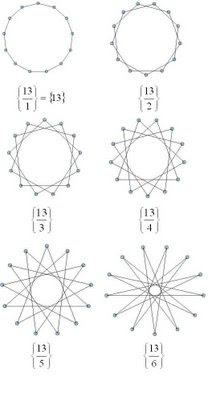Note - by throwing away the unconnected dots in our process we are ignoring star polygons that are made of overlapping disjoint star or regular polygons, for example two overlapping triangles that make a star of David. These also work well with the Schläfli notation. To create these overlapping polygons, if you have any skipped vertices, you just begin your process again beginning with one of the vertices you skipped over. In the case of {6/2}, instead of getting one triangle {3} you will get two overlapping triangles, or 2{3}. To write a program that would draw these you would want to use something more sophisticated than Tinkerplots.

## Tuesday, September 16, 2008

### Extended Multiplication TablesA surprisingly interesting structure is the extended multiplication table, shown above for the numbers seven to ten. The algorithm for drawing these is straight forward - for an n-extended table, start out as if you were writing a "regular" multiplication table, but extend each row so that it gets as close to, without exceeding, n. Another way to think about it is to write out rows of "skip counting up to n" by i for integers i from 1 to n.

This is called an extended multiplication table since it contains a "traditional" multiplication table inside it. The 12-extended table below contains a traditional 3x3 multiplication table.It turns out that 1 appears in an extended table once, and prime numbers appear exactly twice (once in the first column, and once in the first row). In general, for a natural number n, how many times does n appear in the n-extended table?

Before looking at that question, you might want to think about finding easier ways to draw the tables. Drawing out these tables by hand can be tedious - a simple program or spreadsheet might be easier. You can use Fathom, for example, to create the table data and draw it in the collections display. Create a slider m and the attributes listed in the table below (click on the image to see a larger version).Modify the collection display attributes to draw the tables in the collection box. By adding lots of cases and using the slider m to filter out the ones you don't need, you can vary the size of the table easily.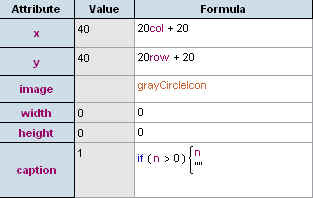Interestingly, the answer to the question "how many times does n appear in the n-extended table?" is the same as the answer to the question posed in a previous post about factor lattices.

# of occurrances of n in the n-extended table = # of nodes in the factor lattice Fn

You can also recast both of these questions (how many occurances of n in the n-extended table, and how many nodesin the Fn factor lattice) as a combinatorial "balls in urns" problem.

Consider a set of colored balls where there are m different colours, where there are ki balls of color i, where i ranges from 1 to m. This would give a total number of balls equal to k1+k2+...+km. Suppose you were to distribute these balls in two urns. How many different distributions would there be? Using some counting techniques, you will find that the answer is (k1+1)*(k2+1)*...*(km+1).

How is this connected to the other problems? Consider the prime factorization of the number. For each prime, choose a colour, and for each occurance of the prime in the factorization, add a new ball of that color. For example for 12 = 3*3*2, choose two colours - say blue=3 and red=2. Since 3 occurs twice and 2 occurs once, there should be two blue balls and one red ball. Now consider distributing these balls in two urns. It turns out that you get (2+1)*(1+1) = 6 possibilities. This is the same number of times 12 occurs in the 12-extended table, and the same number of nodes in the 12-factor lattice. The image below shows the 12-extended table, the 12-factor lattice, and the "ball and urn problem" for the numer 12.For a number n with the prime factorization:The answer to all three questions is given by: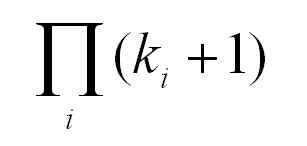## Wednesday, September 10, 2008

### Phyllotaxis Spirals 2The previous post showed some phyllotaxis-like spirals that were created using TinkerPlots. If you bring the same file into Fathom, you can use its support for collection display to create a "graduated" picture that makes the more central seeds look smaller (younger) than the more mature outer seeds.

If you have already created the phyllotaxis data in Fathom, or opened the data created in TinkerPlots in Fathom, you can change the display you get when you drag open a collection box. Under the display tab on the Collection Inspector in Fathom you can set the display properties so that cases no longer show up as uniform gold balls. Setting the display attributes to the values below should give you a growing spiral like the one pictured above.

x = 10x+400
y = 10y+400
image = greyCircleIcon

width = sqrt(r*10)
height = sqrt(r*10)
caption=""

Note tha the x and y that appear on the right-hand side of the equations above are the x and y attributes that you defined for the data, and the x and y that appear on the left-hand correspond to the position of the icons. You can experiment with other formulas for width and height - using a slider to provide a variable instead of the number "10" gives more flexibility.

The images below show some of the other spirals you can obtain by varying the angle between the seeds, as mentioned in the previous post.## Friday, September 5, 2008

### Phyllotaxis SpiralsPhyllotaxis is a term used for the patterns that emerge in the growth of plants. Spiral phyllotaxis is observed in the heads of sunflowers, in pine-cones and pineapples, and in a variety of other plants.

Phyllotaxis is a popular topic in mathematics recreation - it's interesting in its own right and also related to other perennial favorites, Fibonacci numbers and the golden ratio.

The article Modeling Spiral Growth in a GSP Environment describes how to model phyllotaxis-like patterns in GSP. Although GSP works reasonably well, TinkerPlots or Fathom environments seem to be better suited to capturing this particular model - they make the formulas more explicit and easy to manipulate, and they allow for the data generated to be viewed in a variety of ways. The images above were created by porting this model to TinkerPlots.

As the article suggests, experimenting with the the angle between successive seeds allows you to see different resulting patterns - angles that are multiples of rational numbers create patterns of rays while irrational numbers (actually approximate values) give spirals, or spiral/ray combinations (the rays form as the approximation gets more "rational"). A good choice for approximating actual phyllotaxis patterns is to use tau = (1+sqrt(5))/2 in your angle. Here is a listing for the attributes required to generate the pattern in TP or Fathom. The graph/plot is simply the x attribute on the horizontal and the y attribute on the vertical (in TP these need to be fully separated).

n = caseIndex
base_angle = pi*(1+sqrt(5))
r = sqrt(n)

theta = n*base_angle

x = r*cos(theta)
y = r*sin(theta)

The images shown in this post use a collection of 500 cases or "seeds". The base angle is 2pi*tau, and the actual angle for a given seed is a multiple of this base angle.

The model is nice looking and easy to build, but it models only the end result of the growth process, not the process itself. It winds new seeds around the outer edge based on a pre-determined angle. A better model would be one that mirrors what is understood to be the underlying phonomena - new seeds are added to the center and old seeds are pushed out following a set of rules. Under this dynamic method, the angles and spirals are an emergent aspect of the system, rather than the explicit result. This website describes how such a dynamical system could be modeled.

Although the Fathom/TP model does not model the dynamical system that underlies phyllotaxis, it's fun to play with in its own right. You can manually alter the base_angle attribute as suggested by the GSP article. If you add a parameter (a slider) to help you vary the angle, you can obtain a whole family of spiral/ray patterns whose properties you could take a closer look at. Different combinations of angles and sliders will give you various levels of control over the image.

For example, change the formula for base_angle to base_angle = pi*(1+sqrt(5))*base, and create a slider "base". The image below shows the spirals obtained for base = 1...6.Update: Here is an example of how to draw spirals like this in R.

### Factor LatticesThe objects pictured above are interesting structures - they are derived from the prime factorization of a given number n. They can be described in a number of ways - for example, as directed graphs. Because they are nicely structured, they actually form something more special - a lattice. Accordingly, these structures are called factor lattices.

It's easy to start drawing these by hand following the instructions below.

1. The first node is 1
2. Draw arrows out of this node for each of the prime factors of n.
3. The arrows that you just drew should connect to nodes labled with the prime factors of n.

Now, for each of the new nodes that you drew do the following:

4. Start from a node x that is not equal to n.
5. Draw arrows out of this node for each of the prime factors of n/x.
6. The arrows that you just drew (one for each p = n/x) should connect to nodes labled with the numbers p*x.

7. Now repeat 4,5, and 6 for each new node that you have drawn that is not equal to n.

This process is recursive, and ends when you have the complete lattice. The process is well suited for implementation as a computer program - the images above were created using SAGE using output from a Java program based on the algorithm above.

Manually trying out the steps out for a number like n = 24 goes something like this: First write out the prime factorization of 24, 24=(2*2*2)*3 = (2^3)*3. Starting with 1, draw arrows out to 2 and 3. Now looking at each node and following the algorithm, from the 2 you will get arrows out to 4 and 6. From the 3 you will get an arrow out to 6 as well. From 4 you will get arrows out to 8 or 12. From 6 you will get an arrow out to 12 as well. From 8 and from 12 you get arrows out to 24, and you are done.

In general, the algorithm produces a lattice that can be described as follows. Each node is a factor of the given number n. Two nodes are connected by an edge if their prime factorization differs by a single prime number. In other words, if a and b are nodes, and p = b/a, then there is an arrow p:a-->b.

It's a good exercise to make the connections between the lattice structure and the prime factorization of a number n.

1. What does the factor lattice of a prime number look like?
2. If a number is just a power of a prime, what does its lattice look like?
3. If you know the factorization, can you find the number of nodes without drawing the lattice.

The answer to the last question (3) can be expressed as:For example, if n = 24= 2^3*3, then the number of nodes will be (3+1)(1+1) = 8

That these structures can be thought of as "lattices"comes from the fact that you can think of the arrows as an ordering of the nodes, ab. The number 1 is always the least node in the factor lattice for n, while n itself is the greatest node. The property that actually makes these structures a "lattice" is that for any two nodes there is always a lower-bound for any pair of nodes in the lattice, and always an upper-bound for the pair (these are often referred to as meets and joins).

The Wolfram Demonstrations Project has a nice factor lattice demo that will draw factor lattices for a large number of integers for you. There is also a good Wikipedia entry for lattices in general.

## Thursday, August 14, 2008

### Cut-and-Glue Polyhedral ModelsBuilding polyhedral models is a nice way to explore a lot of significant mathematics. The above models were made by printing patterns onto card-stock, cutting them out, and gluing them together. For these models, only triangular faces were used. These can give you a wide variety of cumulated (or augmented) polyhedra. The triangular faces are circumscribed to provide tabs that you glue together. You can fold and glue the tabs so that they are inside the models, but it is easier to leave them out, and they look nice this way (I think).

1. Decide on a model that you would like to make, and figure out how many faces you will need.
2. Copy and paste the images below into a document or presentation slide (PowerPoint works well) for printing. Choose the right ones for your model, and fit as many as you can on a single sheet.
3. Print out onto card-stock. Most desk ink-jet printers can take card-stock instead of printer-paper.
4. Cut out the units, fold the tabs, and assemble and glue.

Throughout this process, it helps if you have pictures of the polyhedra that you want to construct. Poly is a nice software package for browsing through families of polyhedra.

I've found that it works well to bend the tabs using a ruler, that glue-sticks provide the best gluing, and that it helps to hold the model together with binder-clips while assembling.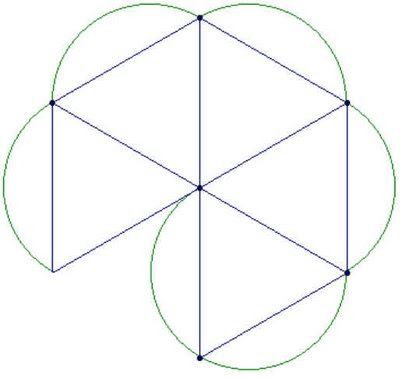## Monday, August 11, 2008

### Polygonal Number FormulasA few of the posts on this blog have dealt with the polygonal numbers. Polygonal numbers are a mainstay of recreational and school mathematics, providing a nice bridge between numbers and shapes. The diagrams above (created using the instructions in an earlier post) show some of the hexagonal numbers.

This post is intended to provide some (limited) background information on two-dimensional polygonal numbers. A previous post suggested an approach for generating a formula for higher-dimensional polygonal numbers. Future posts will elaborate on these numbers some more.

Some examples of two-dimensional polygonal numbers are:

the triangular numbers: 1, 3, 6, 10, 15, ...
the square numbers: 1, 4, 9, 16, 25, ...
the pentagonal numbers: 1, 5, 12, 22, 35,...
the hexagonal numbers: 1, 6, 15, 28, 45, ...

Comparing the listing for the hexagonal numbers with the diagrams above, you can see how the sequences are built diagrammatically. In general, beginning with a single dot, k-sided polygons are built by adding layers (called gnomons) consisting of k-2 segments, with each segment of the gnomon having one more dot than the segments of the previous layer. In this way, the nth gnomon consists of segments each n dots long, but with k-3 dots shared by adjoining segments (the corners).

The description above can lead you to a recursive formula for k-polygonals, writing p_k,n for the nth k-polygonal number: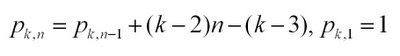Unwinding the recursion gives you a summation formula for k-polygonals:Knowing a little about sums gives you the direct formula for k-polygonals:Coming a little out of left-field is this combinatorial formula for k-polygonals:This last formula expresses two ideas: that the triangular numbers correspond to the r=0 column of Pascal's triangle, and that every polygonal number can be "triangulated":The combinatorial formula for p_kn can be generalized to higher-dimensional polygonal numbers (pyrimidal numbers, etc.). See this previous post for some discussion on this.

The recreation here lies in showing that the various formulas for p_k,n are really the same, and then exploring the relationships between the different k-polygonals. A great resource is J.H. Conway and R.K. Guy's The Book of Numbers.

## Wednesday, August 6, 2008

### Sonobe Phizz DualitySonobe and phizz modular origami units are assembled into polyhedral models in similar ways, but the models that they produce are "dual" to each other (vertices in one correspond to faces in the other). Assembling models out of sonobe and phizz units with this duality in mind provides a nice way of exploring duality and the relationships between edges, faces, and vertices.

The sonobe and phizz modules are both examples of edge modules that come together in groups of 3. In both cases, when the units come together in their groups of 3 they meet in a small triangular pyramid. These pyramids in turn come together in clusters of 3 to 6. The essential difference between the phizz and sonobe modules is in how these clusters form. In the phizz, there is a gap between the groups, so the resulting cluster seems to form the edges of a polygon. In the sonobe, the gap between the groups is small, so the cluster seems to form around a point. Consequently, in the phizz, the center of the cluster takes on the role of a polygonal face, while in the sonobe it is takes on the role of a vertex. Meanwhile, the pyramids formed by the groups of three units become raised vertices in the phizz unit, while in the sonobe they become cumulated faces of the resulting polyhedron.

We naturally interpret what phizz modules generate as polyhedral skeletons, while we see the shapes generated by sonobe as cumulated (or augmented) polyhedra. The fact that this different interpretation is based on the size of the gap that forms in the center of the module clusters suggests that seeing an origami model as a particular polyhedron or its dual is, to some extent, a matter of perception and interpretation.

In phizz models, the fact that the modules come together in groups of 3 dictates that the finished models have vertices of degree (or valence) 3, while in the sonobe case, the 3 units come together to make triangular faces. Dual models have the same number of edges, which corresponds to the number of modules required to build the model.

Some of the models that you can build are summarized below. The picture at the top of the post shows the 30-unit phizz dodecahedron and its dual, the 30-unit sonobe icosahedron.## Thursday, July 31, 2008

### Digit Patterns in Power Sequences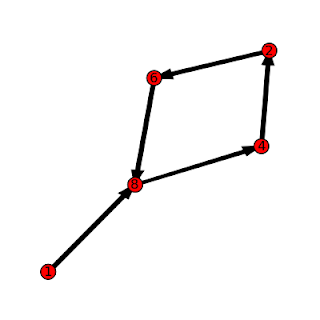Looking at the last few digits that appear in the numbers that form the sequence b^0, b^1, b^2, b^3, ... for b a positive integer, you'll notice that the digits will always begin to repeat after a certain point. For example, looking at the last digit of the sequences for b = 2, 3, and 4 we have the sequences

b = 2: 1, 2, 4, 8, 6, 2, 4, 8, 6, ...
b = 3: 1, 3, 9, 7, 1, 3, 9, 7, ...
b = 4: 1, 4, 6, 4, 6, 4, 6, ...

If we look at the sequence of last two digits of these sequence where b =2 we have

b = 2: 1, 2, 4, 8, 16, 32, 64, 28, 56, 12, 24, 48, 96, 92, 84, 68, 36, 72, 44, 88, 76, 52, 4, ...

This sequence then repeats the loop that began at 4.

We can describe these sequences as T_b,d(n) = (b^n)mod 10^d. Recursively, T_b,d(n) = (T_b,d(n-1)*b)mod 10^d

These sequences are always eventually periodic. Although these sequences are simple to understand and calculate, there are several interesting ways of describing them.

For example, you can think of the elements of T_b,d as a commutative monoid, with multiplication defined as a*b = (a*b)mod 10^d. They form a monoid since 1 is always a member, and you can show that T_b,d is closed under the * operation. It turns out that for some values of b, and d, T_b,d is a group.

You can also think of this set as a finite state machine or graph, where each element is a node and the transition from one node to the next is defined by the operation *b mod 10^d. This provides a nice way of displaying the sequences. The pictures in this post were created by writing a short program to calculate the sequences, and then formatting the output to draw a di-graph in SAGE. The graph at the top of the post is for b=8, d=1, while the graph below is for b=2, d=2. The graph at the bottom of the page is for b=7, d=1.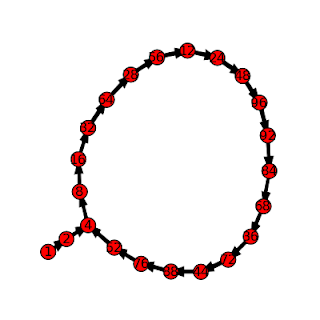## Tuesday, July 22, 2008

### Higher Polygonal Numbers and Pascal's TriangleThe third diagonal column in Pascal's Triangle (r = 2 in the usual way of labeling and numbering) consists of the triangular numbers (1, 3, 6, 10, ...) - numbers that can be arranged in 2-dimensional triangular patterns. The fourth column of Pascal's triangle gives us triangular-based pyramidal numbers (1, 4, 10, 20, ...), built by stacking the triangular numbers. The columns further out give "higher dimensional" triangular numbers that arise from stacking the triangular numbers from the previous dimension.

It is not by coincidence that the triangular and higher-dimensional triangular numbers appear in Pascal's Triangle. If you think about layering of polygonal numbers in terms of equations, you get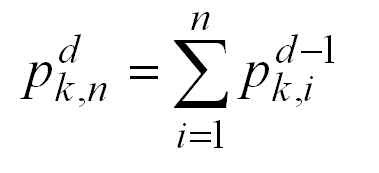In the above equation p^d_(k,n) is the nth k-polygonal number of dimension d. Triangular numbers are the 3-polygonal numbers of dimension 2, square numbers are the 4-polygonal numbers of dimension 2, "square based pyramidal numbers" would be denoted as p^3_(4,n).

From the sum above, you can obtain this equation:Which looks very much like the Pascal Identity C(n,r) = C(n-1,r-1) + C(n-1,r), except for some translation of the variables. To be precise, if we consider the case where k=3 and use r = d and n' = n+d-1 we can translate the triangular numbers into the appropriate positions in Pascal's Triangle.

Along with the definitions for the end columns, the Pascal Identity allows us to generate the whole triangle. This suggests the following strategy for calculating the higher k-Polygonal numbers: create a modified Pascal's Triangle whose first column is equal to k-2 (instead of 1), and whose last column is equal to 1 (as usual). This modified Pascal's Triangle is generated using these initial values and the usual Pascal Identity.

Here is an example with k=5, which sets the first column values equal to 3 (except for the top value, which we keep as 1) and yields the pentagonal numbers (column 3) and the higher pentagonal numbers.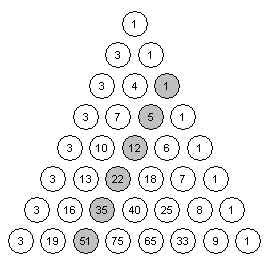The formula for these modified Pascal Triangles is given by this equation: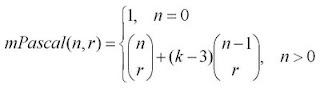If we apply the change of variables mentioned above, we can obtain this general formula for the higher polygonal numbers in terms of combinations: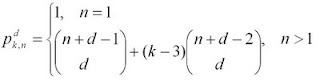This formula illustrates how polygonal numbers are built out of triangular numbers. It says that the nth d-dimensional k-polygonal number is equal to the nth d-dimensional triangular number, plus (k-3) copies of the n-1 d-dimensional triangular number. This is a little easier to understand when you forget about the higher-dimensions and look at the regular 2-dimensional polygonal numbers, as described in another post.